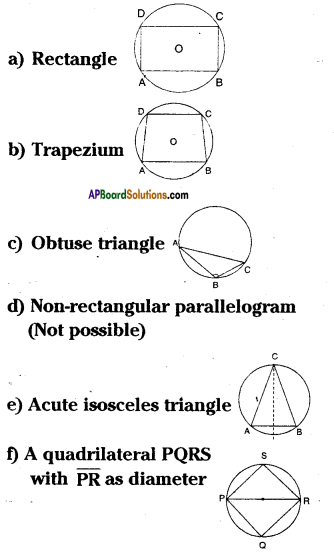# AP Board 9th Class Maths Solutions Chapter 12 Circles Ex 12.5

AP State Syllabus AP Board 9th Class Maths Solutions Chapter 12 Circles Ex 12.5 Textbook Questions and Answers.

## AP State Syllabus 9th Class Maths Solutions 12th Lesson Circles Exercise 12.5Question 1.
Find the values of x and y in the figures given below.
i)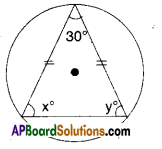Solution:
From the figure x = y [ ∵ angles opp. to opp. to equal sides]
But x + y + 30° = 180°
∴ x + y = 180° – 30° = 150°
⇒ x + y = $$\frac { 150° }{ 2 }$$ = 75°ii)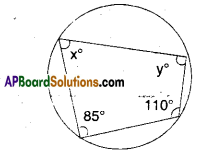Solution:
From the figure x° + 110° = 180°
[ ∵ Opp. angles of a cyclic quad, are supplementary]
y + 85°= 180°
∴ x= 180° – 110°; y = 180° – 85°
x = 70°; y = 95°

iii)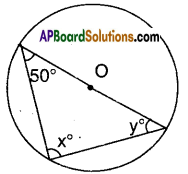Solution:
From the figure x = 90° [angle in a semi-circle]
∴ y = 90° – 50° [∵ angle sum property]
= 40°Question 2.
Given that the vertices A, B, C of a quadrilateral ABCD lie on a circle. Also ∠A + ∠C = 180°, then prove that the vertex D also lie on the same circle.
Solution:
Given : ∠A + ∠C = 180°
∴ ∠B + ∠D = 360° – 180°
[ ∵ sum of the four angles of a quad. is 360 ].
Now in □ABCD, sum of the pairs of opp. angles is 180°.
∴ □ABCD must be a cyclic quadrilateral, i.e., D also lie on the same circle on which the vertices A, B and C lie. Hence proved.

Question 3.
Prove that a cyclic rhombus is a square.
Solution: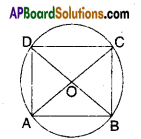Let □ABCD be a cyclic rhombus,
i.e., AB = BC = CD = DA and
∠A + ∠C = ∠B + ∠D = 180°
But a rhombus is basically a parallelo-gram.Question 4.
For each of the following, draw a circle and inscribe the figure given. If a poly¬gon of the given type can’t be in-scribed, write not possible.
a) Rectangle
b) Trapezium .
c) Obtuse triangle
d) Non-rectangular parallelogram
e) Acute isosceles triangle
f) A quadrilateral PQRS with PR as diameter.
Solution: